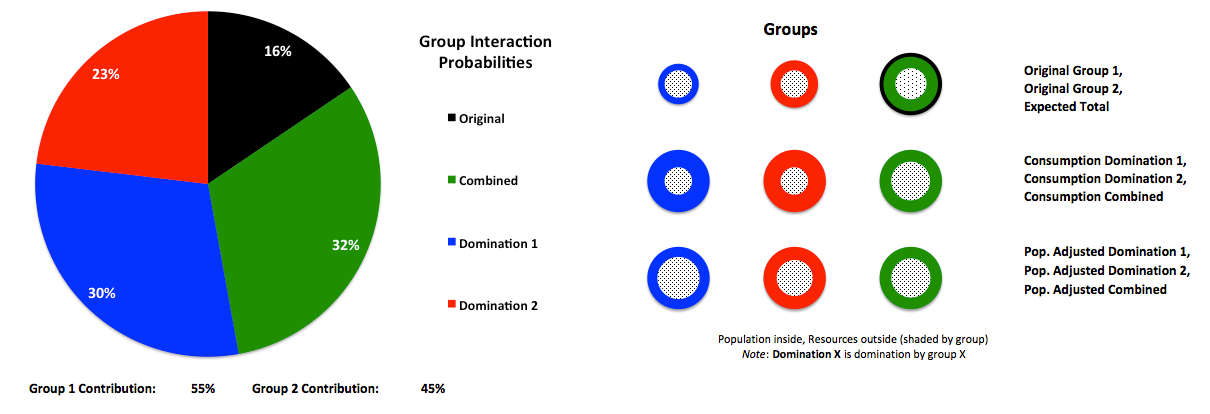# Group Evolution

A group can interact with another group in five ways:

1. No interaction ("original")
2. Take over the other group's resources and distribute resources equally ("consumption domination")
3. Take over the other group and maintain original resources per person by changing population ("pop. adjusted domination")
4. Combine with the other group and distribute resources equally ("consumption combined")
5. Combine with the other group and keep original resources per person by changing population ("pop. adjusted combined")

The group's total preference Pref for each interaction is proportional to its population (P) and the average of the comparisons of resources (R), population, and resources per person (C = R / P):

Pref = P * AVERAGE( Rnew / Roriginal , Pnew / Poriginal , Cnew / Coriginal)

The probability of each interaction is proportional to its preference:

Prob(interaction) = Pref(interaction) / Sum(preferences for all interactions for both groups)

The expected result of all interactions is a group that is the combination of new values based on their probabilities. For example, the expected amount of resources is:

Rexp(group) = SUM[ Prob(interaction) * R(interaction) ]

= Prob(1) * Rnew(1) + Prob(2) * Rnew(2) + Prob(3) * Rnew(3) + Prob(4) * Rnew(4) + Prob(5) * Rnew(5)

The population is:

Pexp(group) = SUM[ Prob(interaction) * P(interaction) ]

Values of both groups add together to form an expected total group:

Rexp(total) = Rexp(group 1) + Rexp(group 2)

Pexp(total) = Pexp(group 1) + Pexp(group 2)

The following diagram shows an example of these relationships.The expected groups [ Rexp(group) , Pexp(group) ] can further interact with each other, or evolve, within the expected total group. The time taken to form a new expected group is called a "generation," with the formation of the first expected group as generation 1. Different variables can also be manipulated to achieve a desired outcome.

For example, global resources and population can be modeled as interactions between two groups: humanity and Nature (the rest of Earth's biosphere). The diagram above represents humanity as Group 1 and Nature as Group 2 after the first generation, and the following diagrams show trajectories of R, P, and C based on free evolution (no manipulation) and the manipulation of two variables for the purpose of maintaining constant human population after the first generation.

Free evolution is denoted by Ev, manipulation of Nature's population is denoted by Np, and manipulation of humanity's resources is denoted by Hr:Of particular interest in this example, here is a closeup of C for humanity:For discussions, see:

© Copyright 2016 Bradley Jarvis. All rights reserved.